BetMGM 2019 Stanley Cup Odds
+750
7.5 to 1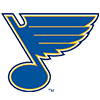Blues
8.4% implied probability

+750
7.5 to 1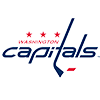Capitals
8.4% implied probability

+800
8 to 1Bruins
7.9% implied probability

+900
9 to 1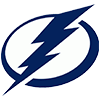Lightning
7.1% implied probability

+1100
11 to 1Penguins
6.0% implied probability

+1100
11 to 1Avalanche
6.0% implied probability

+1200
12 to 1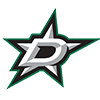Stars
5.5% implied probability

+1500
15 to 1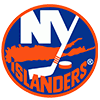Islanders
4.5% implied probability

+1600
16 to 1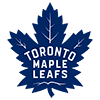Maple Leafs
4.2% implied probability

+1600
16 to 1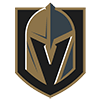Golden Knights
4.2% implied probability

+1700
17 to 1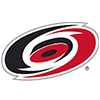Hurricanes
4.0% implied probability

+2000
20 to 1Coyotes
3.4% implied probability

+2000
20 to 1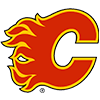Flames
3.4% implied probability

+2000
20 to 1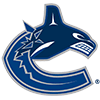Canucks
3.4% implied probability

+2500
25 to 1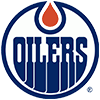Oilers
2.7% implied probability

+2800
28 to 1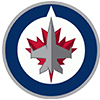Jets
2.5% implied probability

+2800
28 to 1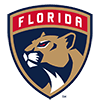Panthers
2.5% implied probability

+3000
30 to 1Predators
2.3% implied probability

+3000
30 to 1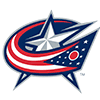Blue Jackets
2.3% implied probability

+3300
33 to 1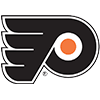Flyers
2.1% implied probability

+4000
40 to 1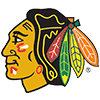Blackhawks
1.7% implied probability

+5000
50 to 1Wild
1.4% implied probability

+5000
50 to 1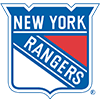Rangers
1.4% implied probability

+5000
50 to 1Sabres
1.4% implied probability

+6000
60 to 1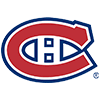Canadiens
1.2% implied probability

+6600
66 to 1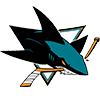Sharks
1.1% implied probability

+25000
250 to 1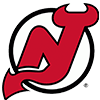Devils
0.3% implied probability

+25000
250 to 1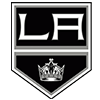Kings
0.3% implied probability

+30000
300 to 1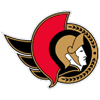Senators
0.2% implied probability

+30000
300 to 1Ducks
0.2% implied probability

+250000
2500 to 1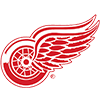Red Wings
0.0% implied probability

No changes have been recorded yet.